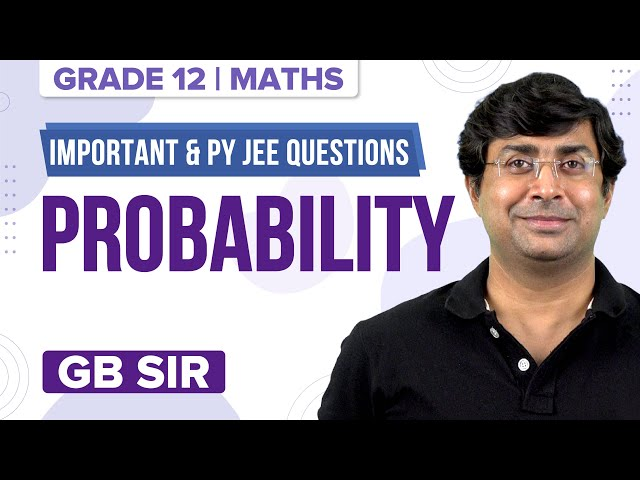Checkout JEE MAINS 2022 Question Paper Analysis : Checkout JEE MAINS 2022 Question Paper Analysis :

# How to Find Conditional Probability for Disjoint Events?

The probability of an event is a measure of the chance of occurrence of an event when an experiment is done. Two events that do not occur at the same time are called disjoint events. These are also known as mutually exclusive events. For example, consider the experiment of rolling a die, a sample space is S = {1, 2, 3, 4, 5, 6}. Let A be an event that an odd number appears and B be an event an even number appears. Here A ⋂ B = φ. A and B are disjoint sets.

In this article, we will learn how to find conditional probability for disjoint events along with the solved problems.

## What is Conditional Probability?

Conditional probability is the probability of one event occurring with some relation to one or more other events. It is denoted by P(A|B). It means the probability of A given that B has already occurred.

### What is meant by Disjoint Events?

Two events that do not occur at the same time are called disjoint events.

## Formula

P(A|B) = [Number of elementary events favourable to A ⋂ B] / [Number of elementary events which are favourable to B]

P(A|B) = P (A ⋂ B) / P(B)

When A and B are disjoint events, P (A ⋂ B) = 0

So P(A|B) = 0.

Let A and B are any two events of a sample space S and F is an event of S such that P(F) ≠ 0

then P((A⋃B) |F) = P(A|F) + P(B|F) – P((A ⋂ B)|F).

If A and B are disjoint events, then P((A⋃B) |F) = P(A|F) + P(B|F).

### Solved Example

Let us understand with the help of an example how to find the conditional probability for disjoint events.

Example: Find the probability that a single toss of a die will result in a number less than 4 if it is given that the toss resulted in an odd number.

Solution:

Let event A be the event that the toss resulted in an odd number and event B be the event that the number is less than 4.

A = { 1,3,5}

B = { 4}

A ⋂ B = {1,3}

P(A ⋂ B) = ⅓

P(B |A) = P(A⋂B)/P(A) = (1/3)/(1/2) = 2/3

To practice more problems on probability, you can click here.

## Probability – Important Topics## Probability – Important Questions### What do you mean by disjoint events in probability?

If two events cannot occur at the same time, then they are called disjoint events. They never happen at the same time.

### If A and B are disjoint sets, what is P(A ⋂ B)?

If A and B are disjoint sets, then P(A ⋂ B) = 0.

### What do you mean by conditional probability?

The probability of occurrence of any event A when another event B in relation to A has already happened is called conditional probability. We denote it by P(A|B). The formula is given by P(A|B) = P (A ⋂ B)/P(B).

Test your Knowledge on Conditional probability